# VBA variables in Excel

In this article we will learn about what are different types of variables used in VBA and how to use them.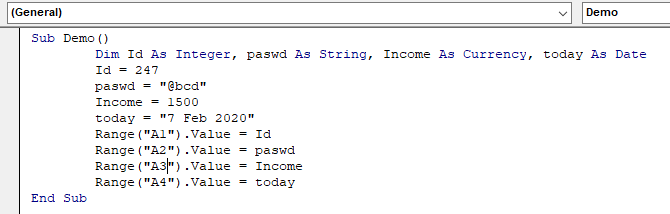What are VBA variables ?

VBA stands for Visual Basic for Applications. It is a programming language from Microsoft. It is used with Microsoft Office applications such as MSExcel, MS-Word and MS-Access whereas VBA variables are specific keywords that are stored in a computer memory or storage system. Later, can be used for value in code and execute. The operator will fetch that value from the system and show it as output. Each variable must be given a name. This article teaches the basics of VBA variables. Each of the sections contain related topics with simple and useful examples.

Some rules for the naming of VBA variables:

1. It's first character must be a letter.
2. It cannot exceed 255 characters in length.
3. Characters like space( ), period( . ), exclamation mark( ! ), @ , & , dollar( \$ ) and hash char (#).
4. It cannot be one of the predefined reserved keywords of VBA.

Syntax:

Sub Demo()

Dim var_name As data_type

End Sub

Numeric data types with value range:

1. Byte ( 0 to 255 )
2. Integer ( -32,768 to 32,767 )
3. Long ( -2,147,483,648 to 2,147,483,648 )
4. Single ( -3.402823E+38 to -1.401298E-45 ) & ( 1.401298E-45 to 3.402823E+38 ).
5. Double ( -1.79769313486232e+308 to -4.94065645841247E-324 ) & ( 4.94065645841247E-324 to 1.79769313486232e+308 ).
6. Currency ( -922,337,203,685,477.5808 to 922,337,203,685,477.5807 )
7. Decimal ( upto 28 decimal places )

Non -numeric data types: variable scope & valid names

1. String ( fixed Length & length range 1 to 65,400 characters )
2. String ( Variable Length & length range 0 to 2 billion characters )
3. Boolean ( either True or False )
4. Date  ( range  January 1, 100 to December 31, 9999 )
5. Object ( Any embedded object )
6. Variant (numeric & Any value as large as Double )
7. Variant ( text & Same as variable-length string )

In VBA, we need to declare the variables before using them by assigning names and data type. In VBA, Variables are either declared Implicitly or Explicitly.

• Implicitly: Below is an example of a variable declared Implicitly.
• label = exceltip.com
• page = 4
• Explicitly: Below is an example of variable declared Explicitly.
• Dim ID As Integer

VBA variable is no different than other programming languages. To declare a variable in VBA you use the keyword "Dim." Let's learn more about commonly used data types considering it one by one.

Integer Data type

First on our list is Integer data type. Integer data type used for the value range from -32,768 to 32,767. For example, If variable name var is set to a value 10. It can be used or recalled whenever required via its var_name.

Sub Demo()

Dim num As Integer

num = 10

range("A1").Value = num

End Sub

Save & run this Demo() code to get the numerical value 10 in the A1 cell of the working worksheet as shown below.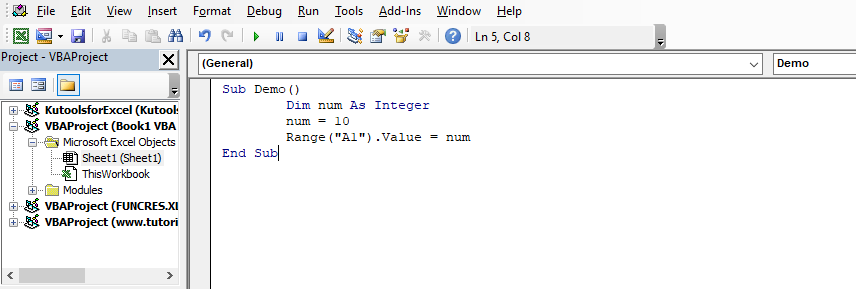Now save this vba workbook.
Open Macro and run this code as shown below.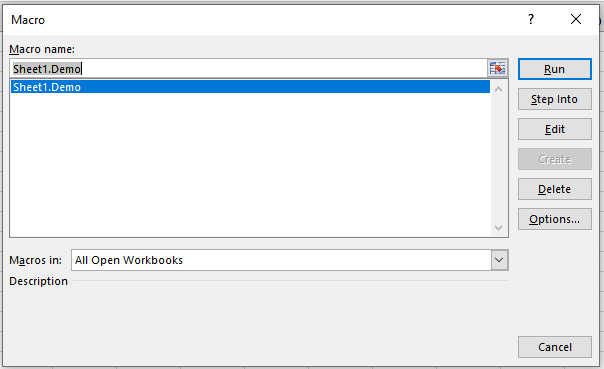Click Run to get the value in A1 cell as 10.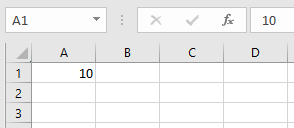As you can see how to use Integer data type and call them, to return a value.

String ( fixed & variable ) Data type

Second on our list is String data type from non - numeric data types. String data type used for the text value character range from 1 to 65,400 characters for fixed & from 0 to 2 billion characters for variable. For example, If variable pasword is set to a value "abcd". It can be used or recalled whenever required via its name.

Sub Demo()

Dim pasword As String

pasword = "abcd"

range("A1").Value = pasword

End Sub

Save & run this Demo() code to get the text value "abcd" in the A1 cell of the working worksheet as shown below.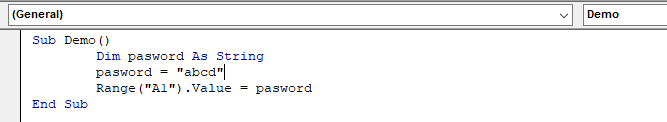Now save this code.
Open Macro and run this code as shown below.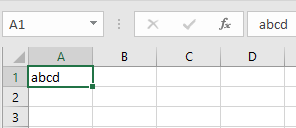Click Run to get the value in A1 cell as 10.As you can see how to use String data type and call them, to return a value.

Currency Data type
Third on our list is currency data type. Currency data type used for the value range from -922,337,203,685,477.5808 to 922,337,203,685,477.5807. For example, If variable loan is set to a value of 10,000. It can be used or recalled whenever required via its var_name.

Sub Demo()

Dim loan As Currency

loan = 10000

range("A1").Value = loan

End Sub

Save & run this Demo() code to get the numerical value 10 in the A1 cell of the working worksheet as shown below.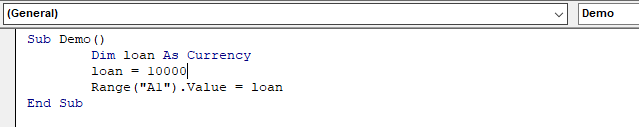Now save this vba workbook.
Open Macro and run this code as shown below.Click Run to get the currency value in A1 cell.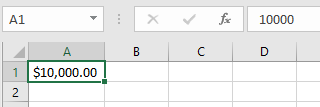As you can see how the code automatically used dollar sign to make the numerical value as currency and how to use currency data type and call them, to return a value.
Date Data type

Fourth common type is Date data type. Date data type used for the date value from January 1, 100 to December 31, 9999. For example, If variable today is set to a date value . It can be used or recalled whenever required via its var_name.

Sub Demo()

Dim today As Date

Date = "7 Feb 2020"

range("A1").Value = Date

End Sub

Save & run this Demo() code to get the date value Febrary 7th, 2020 in the A1 cell of the working worksheet as shown below.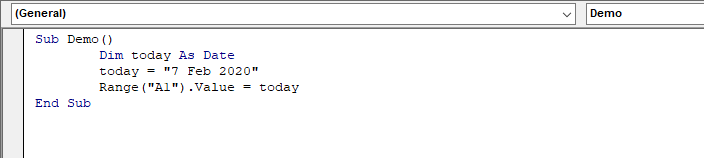Now save this vba workbook.
Open Macro and run this code as shown below.Click Run to get the currency value in A1 cell.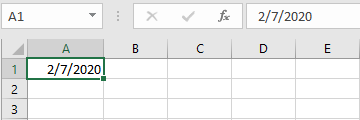As you can see how the code automatically format the cell value to date format to make the numerical value as date and how to use date data type and call them, to return a value.

Example:

The above explained data types some of the most common data types. Now see an example to view as whole subroutine.

Here we will define ID as Integer, paswd as String and Income As currency and Retirement date as Date.Sub Demo()
Dim Id As Integer, paswd As String, Income As Currency, today As Date
Id = 247
paswd = "@bcd"
Income = 1500
today = "7 Feb 2020"
Range("A1").Value = Id
Range("A2").Value = paswd
Range("A3").Value = Income
Range("A4").Value = today
End Sub

Write this code as subroutine and run this Demo() code to get the below result.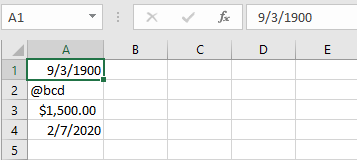As you can see all the values get extracted running the Demo() code.

In all the programming languages, we have variable access specifiers that define from where a defined variable can be accessed. Excel VBA is no Exception. VBA too has scope specifiers. These scope specifiers can be used to set the visibility/scope of a variable in Excel VBA. Learn more about variable scope in vba excel.

Related Articles:

ByRef and ByVal Arguments | When an argument is passed as a ByRef argument to a different sub or function, the reference of the actual variable is sent. Any changes made into the copy of the variable, will reflect in original argument.

Delete sheets without confirmation prompts using VBA in Microsoft Excel | Since you are deleting sheets using VBA, you know what you are doing. You would like to tell Excel not to show this warning and delete the damn sheet.

Add And Save New Workbook Using VBA In Microsoft Excel 2016 | In this code, we first created a reference to a workbook object. And then we initialized it with a new workbook object. The benefit of this approach is that you can do operations on this new workbook easily. Like saving, closing, deleting, etc

Display A Message On The Excel VBA Status Bar | The status bar in excel can be used as a code monitor. When your VBA code is lengthy and you do several tasks using VBA, you often disable the screen update so that you don’t see that screen flickering

Turn Off Warning Messages Using VBA In Microsoft Excel 2016 | This code not only disables VBA alerts but also increases the time efficiency of the code. Let’s see how.

Popular Articles:

50 Excel Shortcuts to Increase Your Productivity | Get faster at your task. These 50 shortcuts will make you work even faster on Excel.

The VLOOKUP Function in Excel | This is one of the most used and popular functions of excel that is used to lookup value from different ranges and sheets.

COUNTIF in Excel 2016 | Count values with conditions using this amazing function. You don't need to filter your data to count specific value. Countif function is essential to prepare your dashboard.

How to Use SUMIF Function in Excel | This is another dashboard essential function. This helps you sum up values on specific conditions.

Terms and Conditions of use

The applications/code on this site are distributed as is and without warranties or liability. In no event shall the owner of the copyrights, or the authors of the applications/code be liable for any loss of profit, any problems or any damage resulting from the use or evaluation of the applications/code.